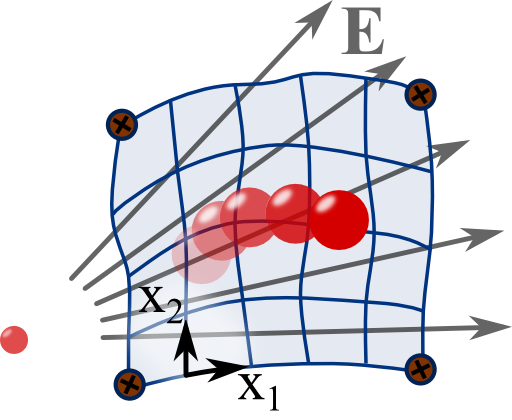## Geodesic Movement of a Charge on a Surface with Electric FieldThe theoretical description of the movement of a particle in some curved 3D-space and the movement of it on a 2D-surface are equivalent. Both entities are described by a metric and the equation of motion is the geodesic equation. Further giving the particle a charge lets it further interact with an electric field. In this problem you can learn to derive the corresponding geodesic equation.## Problem Statement

Consider the movement of a particle with charge $$q$$ and mass $$m$$ attached to a two-dimensional surface $$S$$ in an external electrostatic field. Let us assume that the surface is described by the metric $$g\left(\mathbf{r}\right)=g_{ij}\left(\mathbf{r}\right)dx^{i}dx^{j}$$. Using the definition of the Christoffel symbols, $\Gamma_{kl}^{i}\left(\mathbf{r}\right) \equiv \frac{1}{2}g^{im}\left(\mathbf{r}\right)\left(g_{mk,l}\left(\mathbf{r}\right)+g_{ml,k}\left(\mathbf{r}\right)-g_{kl,m}\left(\mathbf{r}\right)\right)\ ,$find an expression for the equation of motion of the particle. Compare your result to the equations of motion for a free particle in an electric field. Explain the meaning of the arising force terms.

## Relativistic Notation

Note that in this problem it is helpful to use the condensed Einstein notation for which we leave out the summation symbol if an index appears both up- and downwards of a quantity:$\sum_{i}u^{i}v_{i} = u^{i}v_{i}=g_{ij}\left(\mathbf{r}\right)u^{i}v^{i}\ .$As we can see, indices can be “moved” with the metric - lower und upper indices correspond to a representation in cotangent and tangent space, respectively. The length of a vector can be calculated using $$g_{ij}$$: $$\mathbf{u}^{2}=g_{ij}u^{i}u^{j}\equiv g\left(\mathbf{u},\mathbf{u}\right)$$. For a surface in a cartesian (flat) x-y-plane we would simply have $$g_{ij}=\delta_{ij}$$ and the squared length of a vector would be just $$\mathbf{u}^{2}=u_{x}^{2}+u_{y}^{2}$$.
Furthermore, partial derivatives can also be denoted by a comma, $$\partial f\left(x\right)/\partial x=\partial_{x}f\left(x\right)=f_{,x}\left(x\right)$$. See also our section on relativistic electrodynamics for more in-depth informattion.

## Background: Geodesic Motion in General Relativity

In the case of a vanishing electric field we will derive a very general and important result. It explains the motion of a particle not only on surfaces but holds for motions on “generalized surfaces” called manifolds with a given metric $$g_{\mu\nu}\left(x^{\alpha}\right)$$. The equation can also be derived from the requirement of the minimum of the length$L = \int_a^b \sqrt{g_{\mu\nu}\left(x^{\alpha}\left(\tau\right)\right)\frac{dx^{\mu}}{d\tau}\frac{dx^{\nu}}{d\tau}}d\tau$of a curve $$x^{\alpha}\left(\tau\right)$$ connecting two points on that manifold. Such a shortest path is called a geodesic and the equation we will derive consequentially termed geodesic equation.

In general relativity, the metric itself represents the gravitational field. However, it is not the metric of just space but also includes time components. Roughly speaking, all kinds of matter and energy affect the metric as described by the Einstein field equations. In our problem we assumed that the mass or velocity of our charge does not influence the surface, i.e. the metric. In general relativity this is an approximation - the geodesic motion is assumed to not influence the metric. The geodesic equation thus describes the movement of a test particle through spacetime.

Electromagnetic fields itself can be the source of gravitation since they contain energy. This can also be incorporated into general relativity. The corresponding theory is called Einstein-Maxwell theory. In our problem, the metric is given ad-hoc and not influenced by the electrostatic field.